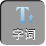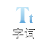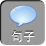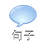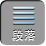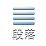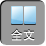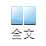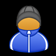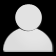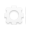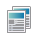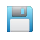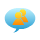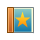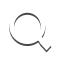-AA+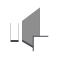1、如图 1所示，在△ ABC中，∠ ACB 90°， BC的垂直平分线 DEBCD，交 ABEFDE上，并且 AFCE.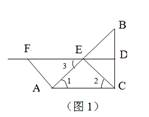DFBCDB= DC.

∴∠ FDB=∠ ACB= 90°.

DFAC.CE= AE=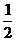AB.

∴∠ 1=∠ 2.

∴∠ 2=∠ 1=∠ 3=∠ F.

∴△ ACE≌△ EFA.

AC= EF.

∴四边形 ACEF是平行四边形.

2、已知：如图 2，在△ ABC中， ABACEAB的中点， DBC上，延长 EDF，使 ED= DF= EB. 连结 FC.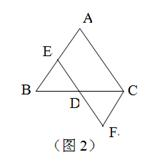ED= EB，∴∠ B=∠ EDB.

∴∠ ACB=∠ EDB. EFAC.

EAB的中点，∴ BD= CD.

∵∠ EDB=∠ FDCED= DF

∴△ EDB≌△ FDC. ∴∠ DEB=∠ F.

ABCF.

∴四边形 AEFC是平行四边形.

3、如图 3，已知平行四边形 ABCD中， EF分别是 ABCD上的点， AE= CFMN分别是 DEBF的中点.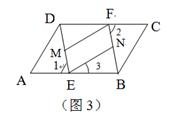∴∠ 1=∠ 2DE= BF.

MN分别是 DEBF的中点，

EM= FN.

DCAB，∴∠ 3=∠ 2.

∴∠ 1=∠ 3. EMFN.

∴四边形 ENFM是平行四边形.

2）四边形 BFDE是平行四边形.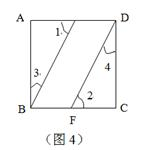AE=ADCF=BC

AE= CF

∴△ ABE≌△ CDF.

2）由（ 1）△ ABE≌△ CDF知，∠ 1=∠ 2，∠ 3=∠ 4.

∴∠ BED=∠ DFB.

∴∠ EBF=∠ EDF.

∴四边形 BFDE是平行四边形.

5、如图 5，在平行四边形 ABCD中， P 1P 2是对角线 BD的三等分点.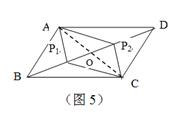∵四边形 ABCD是平行四边形，∴ OA= OCOB= OD.

BP 1= DP 2，∴ OP 1= OP 2.

∴四边形 AP 1 CP 2是平行四边形